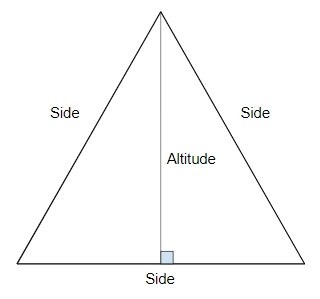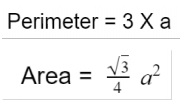# Program to calculate area and perimeter of equilateral triangle in C++

## What is Equilateral Triangle?

As the name suggests, equilateral triangle is the one that have equal sides and also it have equal interior angles of 60° each. It is also known as regular triangle because it’s a regularpolygon

Properties of equilateral triangle are −

• 3 sides of equal length
• Interior angles of same degree which is 60

Given below is the figure of equilateral triangle## Problem

Given with the side of equilateral triangle the task is to find the area and perimeter of a triangle where area is the space occupied by the shape and perimeter is the space occupied by its boundaries.

To calculate area and perimeter of a equilateral triangle there is a formula## Example

Input-: side=14.0
Output-: Area of Equilateral Triangle is : 84.8705
Perimeter of Equilateral Triangle: 42

## ALGORITHM

Start
Step 1 -> Declare function to calculate area of equilateral trainagle
Float area(float side)
Return sqrt(3) / 4 * side * side
Step 2 -> Declare function to calculate perimeter of equilateral trainagle
Float perimeter(float side)
Return 3 * side
Step 3 -> In main()
float side = 14.0
call area(side)
call perimeter(side)
Stop

## CODE

Live Demo

#include <bits/stdc++.h>
using namespace std;
//function to calculate area of equilateral triangle
float area(float side){
return sqrt(3) / 4 * side * side;
}
//function to calculate perimeter of equilateral triangle
float perimeter(float side){
return 3 * side;
}
int main(){
float side = 14.0;
cout << "Area of Equilateral Triangle is : "<<area(side);
cout << "\nPerimeter of Equilateral Triangle: "<<perimeter(side);
return 0;
}

## Output

Area of Equilateral Triangle is : 84.8705
Perimeter of Equilateral Triangle: 42